MathematicsSolving Singular BVPs

The function `bvp4c` solves a class of singular BVPs of the form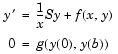(5-2)

It can also accommodate unknown parameters for problems of the form

•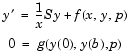Singular problems must be posed on an interval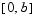with 0 -->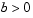. Use `bvpset` to pass the constant matrix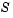to `bvp4c` as the value of the `'SingularTerm'` integration property. Boundary conditions at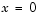must be consistent with the necessary condition for a smooth solution,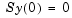. An initial guess should also satisfy this necessary condition.

When you solve a singular BVP using

• ```sol = bvp4c(@odefun,@bcfun,solinit,options)
```

`bvp4c` requires that your function `odefun(x,y)` return only the value of the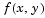term in Equation 5-2.

Example: Solving a BVP that Has a Singular Term

Emden's equation arises in modeling a spherical body of gas. The PDE of the model is reduced by symmetry to the ODE

•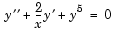on an interval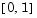. The coefficient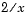is singular at, but symmetry implies the boundary condition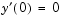. With this boundary condition, the term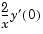is well-defined as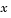approaches 0. For the boundary condition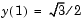, this BVP has the analytical solution

•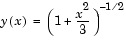Note    The demo `emdenbvp` contains the complete code for this example. The demo uses subfunctions to place all required functions in a single M-file. To run this example type `emdenbvp` at the command line. See BVP Solver Basic Syntax and Solving BVP Problems for more information.

1. Rewrite the problem as a first-order system and identify the singular term. Using a substitution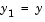and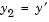, write the differential equation as a system of two first-order equations
•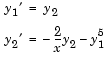1. The boundary conditions become

•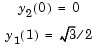1. Writing the ODE system in a vector-matrix form

•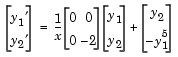1. the terms of Equation 5-2 are identified as

•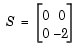1. and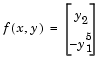1. Code the ODE and boundary condition functions. Code the differential equation and the boundary conditions as functions that `bvp4c` can use.
• ```function dydx = emdenode(x,y)
dydx = [  y(2)
-y(1)^5 ];
function res = emdenbc(ya,yb)
res = [ ya(2)
yb(1) - sqrt(3)/2 ];
```
2. Setup integration properties. Use the matrix as the value of the `'SingularTerm'` integration property.
• ```S = [0,0;0,-2];
options = bvpset('SingularTerm',S);
```
3. Create an initial guess. This example starts with a mesh of five points and a constant guess for the solution.
•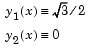1. Use `bvpinit` to form the guess structure

• ```guess = [sqrt(3)/2;0];
solinit = bvpinit(linspace(0,1,5),guess);
```
1. Solve the problem. Use the standard `bvp4c` syntax to solve the problem.
• ```sol = bvp4c(@emdenode,@emdenbc,solinit,options);
```
2. View the results. This problem has an analytical solution
•1. The example evaluates the analytical solution at 100 equally-spaced points and plots it along with the numerical solution computed using `bvp4c`.

• ```x = linspace(0,1);
truy = 1 ./ sqrt(1 + (x.^2)/3);
plot(x,truy,sol.x,sol.y(1,:),'ro');
title('Emden problem -- BVP with singular term.')
legend('Analytical','Computed');
xlabel('x');
ylabel('solution y');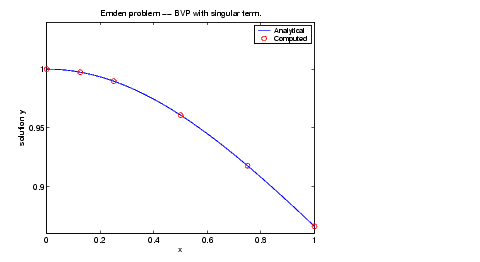```Using Continuation to Make a Good Initial Guess Solving Multi-Point BVPs© 1994-2005 The MathWorks, Inc.